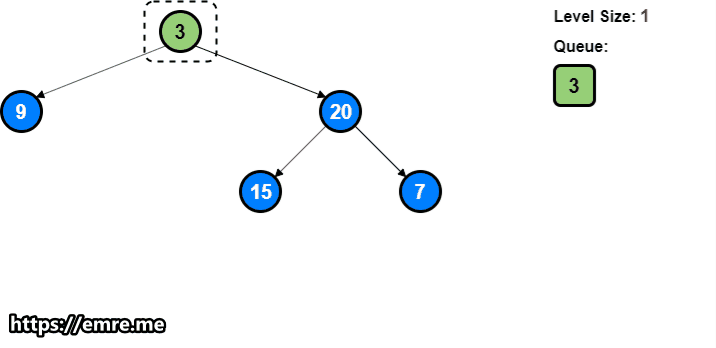# Coding Patterns: Breadth First Search (BFS)

In Coding Patterns series, we will try to recognize common patterns underlying behind each algorithm question, using real examples from Leetcode.

Previous posts were about Sliding Window, Two Pointers, Fast & Slow Pointers, Merge Intervals, Cyclic Sort and In-place Reversal of a Linked List patterns and today, we will introduce Breadth First Search (BFS) pattern which is very useful to solve the problems involving traversal of a tree in a level-by-level order.

We will use a Queue to keep track of all the nodes of a level before we jump onto the next level. This also means that the space complexity of the algorithm will be O(N), where N is the maximum number of nodes on any level.

## Problem: Binary Tree Level Order Traversal

LeetCode 102 - Binary Tree Level Order Traversal [medium]

Given a binary tree, return the level order traversal of its nodes’ values. (ie, from left to right, level by level).

For example:

Given binary tree: `[3, 9, 20, null, null, 15, 7]`

``````    3
/ \
9  20
/  \
15   7
``````

return its level order traversal as:

``````[
,
[9,20],
[15,7]
]
``````

Since we need to traverse all nodes of each level before moving onto the next level, we can use the Breadth First Search (BFS) technique to solve this problem.

We can use a Queue to efficiently traverse in BFS fashion. Here are the steps of our algorithm:

1. Start by pushing the `root` node to the queue.
2. Keep iterating until the queue is empty.
3. In each iteration, first count the elements in the queue (let’s call it `level_size`). We will have these many nodes in the current level.
4. Next, remove `level_size` nodes from the queue and push their `value` in an array to represent the current level.
5. After removing each node from the queue, insert both of its children into the queue.
6. If the queue is not empty, repeat from step 3 for the next level.``````from collections import deque
from typing import List

class TreeNode:
def __init__(self, x):
self.val = x
self.left = None
self.right = None

class Solution:
def levelOrder(self, root: TreeNode) -> List[List[int]]:
result = []
if root is None:
return result

queue = deque()
queue.append(root)
while queue:
level_size = len(queue)
current_level = []
for _ in range(level_size):
current_node = queue.popleft()
current_level.append(current_node.val)  # add node to current level

if current_node.left:
queue.append(current_node.left)
if current_node.right:
queue.append(current_node.right)
result.append(current_level)
return result
``````

Time Complexity: O(N) where `N` is the total number of nodes in the tree.

Space Complexity: O(N), since we need an O(N) space to return the result. We will also need O(N) for the queue.

## How to identify?

This approach is quite useful when dealing with the problems involving traversal of a tree in a level-by-level order.

When the problem asks the traversal of a tree, you should think about Breadth First Search (BFS) pattern and using it in combination with the Queue structure.

Tags:

Categories:

Updated: